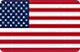# Liquidation risk calculator

• Risk of falling from peak
• Bankruptcy risk

# Risk of Ruin Calculator

The risk of ruin in forex is the chance that a trader would lose a significant amount of money in their trading and investment activities, to the point where it is impossible to recover from the past losses or continue trading. Be aware that this is not your entire account balance, as you should never put your entire savings on the line.
As a mathematical model, the Risk of Ruin (RoR) simulates the probability of losing a trading account's whole balance depending on the winning/losing ratio and the amount of money each trade. Ideally, you should never have a drawdown of 50% or less, since if you do, you'll need a 100% return in order to break even.
Formula:
Quite a few methods to calculate the risk of ruin, but the most commonly use formula is as follows:
Risk of ruin = (1 - (B - P)) / (1 + (B - P)) ^ U
B = Probability of winning (Ex. 0.6 for 60%)
P = Probability of losing (Ex. 0.4 for 40%)
^ U = Power of the maximum number of consecutives losing trades before ruin.
Example:
Let’s say Mike has a \$50,000 account and is willing to risk a maximum drawdown of 30%, which is -\$15,000. Suppose he has proven by his transactions that he can obtain the following averages: profit = 60%, loss = 40%, risk per transaction 1% of the account (\$500) so the maximum number of trades he can take and lose consecutively is 30 trades before he reaches the ruin point (maximum drawdown of 30%).
His risk of ruin is therefore calculated as follows:
(1-(0.2)/1+(0.2)) ^30 = (0,666666)^30 = 0,000005214 = 0 % (rounded down to the inferior number).
Thus, he can take risks without fear of failure because of the low likelihood of financial disaster. That's assuming, of course, that he maintains his current trading practices. The likelihood of going bankrupt might rise or fall depending on how the profit margin and profit/loss ratio change over time.
While another trader, Mary, takes too much risk and is undercapitalized. She has an account of \$10,000 and is willing to risk a maximum Drawdown of 30%, which is a ruin point at -\$3,000. Profit = 60%, Loss = 40%, risk per trade 10% of the account (-\$1,000) so the maximum number of trades she can make and lose consecutively is 3 trades before she reaches her point of ruin (Maximum drawdown of 30%).
Her risk of ruin is therefore calculated as follows:
(1-(0.2)/1+(0.2))^3 =(0,666666)^3 = 0,2962954074083 = 30% (rounded up to the nearest number)
This is a considerable difference, despite the fact that she has a smaller account and is consequently more vulnerable to losing money. As a result, the likelihood of Mary going bankrupt is significant.
Select Country/DistrictUnited States※ The content of this website abides with local laws and regulations.You are visiting the WikiFX website. WikiFX Internet and its mobile products are an enterprise information searching tool for global users. When using WikiFX products, users should consciously abide by the relevant laws and regulations of the country and region where they are located.
consumer hotline：006531290538
Official Email：support@wikifx.com；
Mobile Phone Number：234 706 777 7762；61 449895363
Telegram：+60 103342306
Whatsapp：+852-6613 1970；+44-7517747077